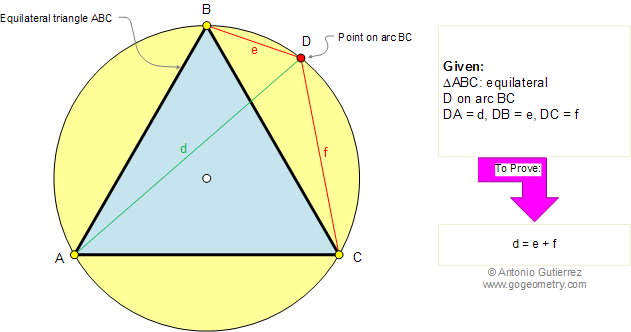Problem 256. Equilateral Triangle, Circumcircle, Point, Vertices, Distances. Level: High School, College, SAT Prep. The figure shows an equilateral triangle ABC inscribed in a circle. If a point D is on arc BC, DA = d, DB = e, and DC = f, prove that d = e + f. View or post a solutionSee also: GeoGebra of problem 256 Recent Additions